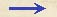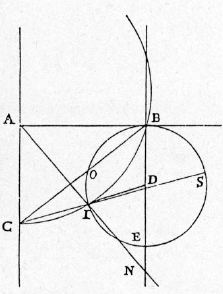Discorsi Propositions 2/35-pr-14-lemma-3 Discorsi Proposition2/35-pr-14-lemma-3{20} LEMMA LEMMASit circuli quadrans ACIB; et ex B, ipsi AC parallela, BE; et ex quovis centro in ea sumpto circulus BOES descriptus, tangens AB in B, et secans {261} circumferentiam quadrantis in I; et iuncta sit CB, et CI usque ad S extensa: dico, lineam CI minorem semper esse ipsa CO. Let ACIB be the quadrant of a circle; from B draw BE parallel to AC; about any point in the line BE describe a circle BOES, touching AB at B and intersecting the circumference of the quadrant at I. Join the points C and B; draw the line CI, prolonging it to S. Then, I say, the line CI is always less than CO. Iungatur AI, quae circulum BOE tanget. Si enim ducatur DI, erit aequalis ipsi DB; cum vero DB quadrantem tangat, tanget etiam eumdem DI et ad diametrum AI erit perpendicularis; quare et ipsa AI circulum BOE tanget in I. Et quia angulus AIC maior est {10} angulo ABC, cum maiori insistat peripheriae, ergo angulus quoque SIN ipso ABC maior erit: quare portio IES maior est portione BO, et linea CS, centro vicinior, maior ipsa CB: quare et CO maior CI, cum SC ad CB sit ut OC ad CI. Idem autem magis accidet, si (ut in altera figura) BIC quadrante fuerit minor. Nam perpendicularis DB circulum secabit CIB; quare DI quoque, cum ipsi {20} DB sit aequalis; et angulus DIA erit obtusus, et ideo AIN circulum quoque BIE secabit. Cumque angulus ABC minor sit angulo AIC, qui aequatur ipsi SIN; iste autem est adhuc minor eo qui ad contactum in I fieret per lineam SI; ergo portio SEI est longe maior portione BO: unde etc. Quod erat demonstrandum. Draw the line AI touching the circle BOE. Then, {261} if the line DI be drawn, it will be equal to DB; but, since DB touches the quadrant, DI will also be tangent to it and will be at right angles to AI; thus AI touches the circle BOE at I. And since the angle AIC is greater than the angle ABC, subtending as it does a larger arc, it follows that the angle SIN is also greater than the angle ABC. Wherefore the arc IES is greater than the arc BO, and the line CS, being nearer the center, is longer than CB. Consequently CO is greater than CI, since SC:CB = OC:CI. This result would be all the more marked as in the second figure, the arc BIC were less than a quadrant. For the perpendicular DB would then cut the circle CIB; and so also would DI which is equal to BD; the angle DIA would be obtuse and therefore the line AIN would cut the circle BIE.Since the angle ABC is less than the angle AIC, which is equal to SIN, and still less than the angle which the tangent at I would make with the line SI, it follows that the arc SEI is far greater than the arc BO; whence, etc. Q. E. D.Discorsi Propositions 2/35-pr-14-lemma-3 Discorsi Proposition2/35-pr-14-lemma-3